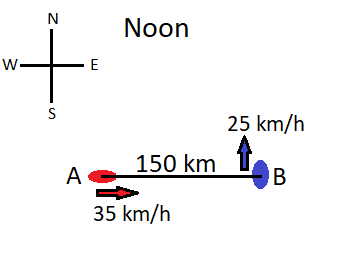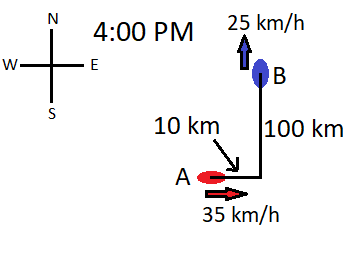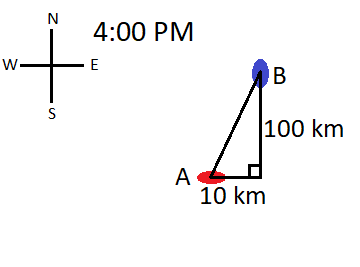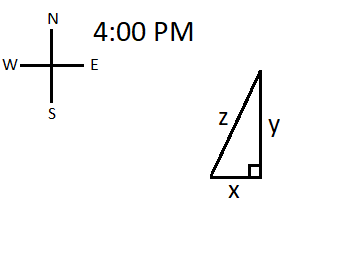## RELATED RATES – 4 Simple Steps

Related rates problems are one of the most common types of problems that are built around implicit differentiation and derivatives.  Typically when you’re dealing with a related rates problem, it will be a word problem describing some real world situation.

Typically related rates problems will follow a similar pattern.  They can usually be broken down into the following four related rates steps:

• The first thing you will usually want to do after reading the problem is to draw a sketch of the situation being described.
• Then you will need to come up with some equation that relates the different quantities described to you, which may be volumes, areas, or distances.
• Once you have this equation, you’ll perform implicit differentiation on both sides of the equation, usually with respect to time.
• Then you just need to solve for the desired rate of change that the question is asking about.

I always think the best way to learn a new concept is practice, practice, practice.  So let’s jump into an example.  If you want to skip ahead, there is a list of other examples at the bottom of this page with a link to their solutions.

## Example 1

At noon, ship A is 150 km west of ship B.  Ship A is sailing east at 35 km/h and ship B is sailing north at 25 km/h.  How fast is the distance between the ships changing at 4:00 PM?

### 1. Draw a sketch

Like I said before, the best thing to do first is draw a picture of what is being described.  The problem tells us where these ships are, and the direction and speed at which they’re moving at noon, so I think we should start by drawing our scene at noon, seen in Figure 2.1.Figure 2.1

Now let’s think about what will be happening between noon and 4:00 PM.  We know the speed and direction both of these ships are traveling.  We also know that they will be moving for 4 hours before we consider their position again.

Ship A:  This ship is moving 35 km/h for 4 hours.  Therefore, between noon and 4:00 PM we know it will move east

$$35\frac{km}{h}*4h=140km.$$

Ship B:  This ship is moving 25 km/h for 4 hours.  Therefore, between noon and 4:00 PM we know it will move north

$$25\frac{km}{h}*4h=100km.$$

Considering both of these facts, at 4:00 PM, our scene would look something like this:Figure 2.2

### 2. Come up with your equation

Now, the next thing we need to do is come up with an equation that relates the different distances given based on where the boats are at 4:00 PM.  We also want to consider what the question is asking before we come up with this equation.  The question is asking us to find how fast the distance between the boats is changing.  We won’t be able to find an equation for this right away, but this tells us that we need an equation that involves the distance between the two boats.  From there, we can figure out how fast that distance is changing.

To do this, we will want to think of the drawing in Figure 2.2 as a triangle.  The three vertices of the triangle would be ship A, ship B, and the point in the water where boat B was at noon, which is shown in the drawing above.  Doing this gives us something like this:Figure 2.3

It is important to remember that both ships are still moving, they don’t stop at 4:00.  As a result of this, the sides of our triangle are not constants.  Instead, they would be variables, so the triangle we may want to consider is this one:Figure 2.4

From this, we can create our equation.  What we want to think about is what variable has to do with the value the question is asking us to find and what variables do we know something about.

What are we looking for?

The question asks us to find how fast the distance between the ships is changing.  By comparing Figure 2.4 to the prior drawings, we can see that side $$z$$ is the one that represents the distance between the two ships.  Therefore, we will need to include $$z$$ in our equation which we will eventually differentiate.

What do we know about?

This is where we want to consider the actual numerical values we have figured out and how they relate to the different variables in our drawing.  At 4:00 PM we know the values of $$x$$ and $$y$$.  We also know the speed at which the ships are moving in relation to a fixed point, which is the vertex of the triangle that makes up the right angle.  Therefore, we not only know the values of $$x$$ and $$y$$, but we also know their rates of change.  These will simply be the speeds of the ships.

Putting it into an equation.

We need an equation that relates the thing we are looking for with the things we already know.  Since we’re looking for some information about $$z$$ (the rate of change of $$z$$), and we know everything about $$x$$, $$y$$, and both of their rates of change at 4:00, we need an equation relating $$x$$, $$y$$, and $$z$$.

Since we know the relationship between these variables is that they are the side lengths of a right triangle, the simplest equation we can use is Pythagorean Theorem.  Based on this we know that

$$z^2=x^2+y^2.$$

### 3. Implicit differentiation

Now that we have our equation, we need to take its derivative.  This is where the implicit differentiation comes in.  Before we do this, let’s think about what we want to differentiate with respect to.

I mentioned in the beginning of this article that we will usually differentiate with respect to time.  The reason for this is that $$x$$, $$y$$, and $$z$$ are all changing as time passes.  If fact, since we know the ships’ initial positions and their velocities, we could actually write $$x$$, $$y$$, and $$z$$ as functions of time.  Therefore, when we differentiate both sides of our equation, we will treat $$x$$, $$y$$, and $$z$$ as functions, and time (represented by $$t$$) will be the variable.  If you want a bit more explanation on the next few step, I explained this a bit more here.

$$z^2=x^2+y^2$$

$$\frac{d}{dt}\big[ z^2\big]=\frac{d}{dt}\big[ x^2+y^2\big]$$

$$2z\frac{dz}{dt}=2x\frac{dx}{dt}+2y\frac{dy}{dt}$$

Now we can divide both sides by $$2$$ to simplify.

$$z\frac{dz}{dt}=x\frac{dx}{dt}+y\frac{dy}{dt}$$

### 4. Solve for desired rate of change

Now remember, the thing we are trying to find in this problem is the rate of change of $$z$$.  This is exactly what $$\frac{dz}{dt}$$ represents, so we will solve for this by dividing both sides by $$z$$.

$$\frac{dz}{dt}=\frac{x\frac{dx}{dt}+y\frac{dy}{dt}}{z}$$

All we have left to do now is plug in all the pieces on the right side of the equation and that would give us our answer.  We are looking for the value of $$\frac{dz}{dt}$$ at 4:00 PM, so we need to use the values of all the other variables based on what they are at 4:00 PM also.  We can gather most of the information we need from Figure 2.2, shown above.  Here it is again:Figure 2.2

Clearly, we can see that $$x=10km$$ and $$y=100km$$ at 4:00.  We also know the value of $$\frac{dx}{dt}$$ and $$\frac{dy}{dt}$$. The reason for this is that these two things represent the rate of change of $$x$$ and $$y$$.  Since $$x$$ and $$y$$ represent the distance between each of the ships and a fixed point, $$\frac{dx}{dt}$$ and $$\frac{dy}{dt}$$ would be given by the speed of each ship.  It is also important to point out that is works because the ships are moving directly toward the fixed point or directly away from the fixed point.  The length of $$x$$ is shrinking by $$35\frac{km}{h}$$ because ship A is moving at that speed.  Therefore, we know

$$\frac{dx}{dt}=-35\frac{km}{h}.$$

Notice this value is negative.  This is simply because $$x$$ is getting smaller at 4:00.  Similarly, we know that $$y$$ is getting larger by $$25\frac{km}{h}$$ at 4:00 and therefore we can say that

$$\frac{dy}{dt}=25\frac{km}{h}.$$

Now the only thing we still need to figure out is the value of $$z$$ at 4:00 PM.  To do this, let’s go back to our Pythagorean Theorem equation we looked at earlier.  We know

$$z^2=x^2+y^2.$$

Since we already know the values of $$x$$ and $$y$$ at 4:00, we can just plug them into the Pythagorean Theorem equation to get the value of $$z$$ at 4:00.

$$z^2=10^2+100^2$$

$$z^2=100+10,000$$

$$z^2=10,100$$

$$z=\sqrt{10,100}$$

Now that we have all the pieces figured out, we can go back and plug them into our equation for $$\frac{dz}{dt}$$.

$$\frac{dz}{dt}=\frac{10*(-35)+100*25}{\sqrt{10,100}}$$

$$\frac{dz}{dt}=\frac{2,150}{\sqrt{10,100}}\approx 21.393\frac{km}{h}$$

So we can say that the distance between the two ships is changing at about $$21.393\frac{km}{h}$$ at 4:00 PM.

## Other Examples

Like I said before, the best way to gain an understanding of related rates problems is practice.  Here are some more complete solutions of other fun related rates problems.  Just click on the problem to see the full solution.

#### Triangles

A kite 100 ft above the ground moves horizontally at a speed of 8 ft/s. At what rate is the angle between the string and the horizontal decreasing when 200 ft of string has been let out?

A plane flies horizontally at an altitude of 5 km and passes directly over a tracking telescope on the ground. When the angle of elevation is pi/3, this angle is decreasing at a rate of pi/6 rad/min. How fast is the plane traveling at this time?

The top of a ladder slides down a vertical wall at a rate of 0.15 m/s. At the moment when the bottom of the ladder is 3 m from the wall, it slides away from the wall at a rate of 0.2 m/s. How long is the ladder?

#### Squares

Each side of a square is increasing at a rate of 6 cm/s. At what rate is the area of the square increasing when the area of the square is 16 cm^2?

#### Cones

Water is leaking out of an inverted conical tank at a rate of 10,000 cm^3/min at the same time water is being pumped into the tank at a constant rate. The tank has a height 6 m and the diameter at the top is 4 m. If the water level is rising at a rate of 20 cm/min when the height of the water is 2 m, find the rate at which water is being pumped into the tank.

#### Spheres

If a snowball melts so that its surface area decreases at a rate of 1 cm^2/min, find the rate at which the diameter decreases when the diameter is 10 cm.

The radius of a sphere is increasing at a rate of 4 mm/s. How fast is the volume increasing when the diameter is 80 mm?

#### Cylinders

A cylindrical tank with radius 5 m is being ﬁlled with water at a rate of 3 m^3/min. How fast is the height of the water increasing?

As always, I’d love to see your questions!  You can leave a comment below or email your questions to me at jakesmathlessons@gmail.com.  Whether you have questions about this lesson or want me to post a solution for another problem you’re stuck on, just send me a message.